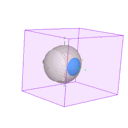3D Dose Matrix

Isodose surface calculations can add significantly to dose calculation and 3D rendering time. Since isodose surfaces are not necessary for routine treatment planning, the 3D matrix is not calculated automatically. In PS6, 3D calculations are run on a separate thread from the main program. Progress is updated in the Setup window status line below the toolbar. You may continue to use PS while 3D calculations are in progress but cannot change anything that would affect the dose calculations.

Each plaque includes its own a 3D matrix data structure. The 3D matrix bounds are 20 mm lateral to the plaque's central axis (CAX), 5 mm behind the plaque, and 40 mm in front of the plaque. Dose outside these bounds is assumed to be zero. The 3D matrix has nonuniform density. The spacing between calculation points varies between 0.5 and 1.0 mm laterally and between 0.25 and 2.0 mm in front of (and behind) the plaque with the greatest calculation point density at the plaque face. The matrix size (and hence calculation speed) can be customized from the Plaque 3D Dose Matrix Menu.

Illustrated below are the matrix bounds and the 85 Gy isodose surface of a BEBIG CCB plaque.

•• Matrix bounds

•• Isodose surface